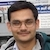# NAEST 2014 Screening Test: Pressure in Connected BottlesBy

Two bottles, say bottle 1 and bottle 2, are connected with a pipe near their bottom. Initially, bottle 1 is partially filled with water, bottle 2 is completely empty and caps are fitted on both of them. Then, the cap of bottle 2 is removed. The atmospheric pressure is $p_0$.

Question 1: The pressure of air in the bottle 1 is

1. less than $p_0$
2. more than $p_0$
3. equal to $p_0$

Question 2: The pressure of air in the bottle 2 is

1. less than $p_0$
2. more than $p_0$
3. equal to $p_0$

Question 3: If cap from the bottle 1 is also removed then

1. no water will flow.
2. some water will flow from botttle 1 to bottle 2.
3. water will be equally divided in both bottles.

Solution: The water is not flowing from the bottle 1 to the bottle 2 when both bottles are capped. This is possible when pressure on both ends of the pipe are equal.When the cap of bottle 2 is removed, the air inside it gets connected to the atmospheric air. The air pressure in bottle 2 becomes equal to the atmospheric pressure. The water pressure in bottle 1 at level of the pipe is also equal to $p_0$ (because neither water nor air moves from one bottle to the other). If the height of the water column (above the pipe) is $h$ then the pressure of air in bottle 1 is given by \begin{align} p_1=p_0-\rho g h < p_0 \end{align} Note that $\rho$ is the density of water and $\rho g h$ is the hydrostatic pressure due to the water column of height $h$.

When the cap of bottle 1 is also opened, the air inside it gets connected with the atmosphere. The pressure just above the water surface is $p_0$ and pressure at the level of the pipe becomes equal to $p_0+\rho g h$. There is a pressure difference between the two ends of the pipe. This pressure difference makes the water to flow from bottle 1 to the bottle 2. The flow continues until pressure difference at two ends of the pipe becomes equal. This condition occurs when the height of water level in both the bottles is the same. Thus, water will be equally divided in both bottles.

Can you guess a process by which the initial condition of this experiment is achieved?